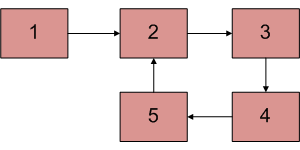# C++ Program For Checking Linked List With A Loop Is Palindrome Or Not

• Last Updated : 06 Jan, 2022

Given a linked list with a loop, the task is to find whether it is palindrome or not. You are not allowed to remove the loop.Examples:

```Input: 1 -> 2 -> 3 -> 2
/|      |/
------- 1
Output: Palindrome
Linked list is 1 2 3 2 1 which is a
palindrome.

Input: 1 -> 2 -> 3 -> 4
/|      |/
------- 1
Output: Not Palindrome
Linked list is 1 2 3 4 1 which is a
not palindrome. ```

Algorithm:

1. Detect the loop using the Floyd Cycle Detection Algorithm.
2. Then find the starting node of the loop as discussed in this.
3. Check the linked list is palindrome or not as discussed in this.

Below is the implementation.

## C++

 `// C++ program to check if a linked list  ` `// with loop is palindrome or not. ` `#include ` `using` `namespace` `std; ` ` `  `// Link list node  ` `struct` `Node ` `{ ` `    ``int` `data; ` `    ``struct` `Node * next; ` `}; ` ` `  `/* Function to find loop starting node. ` `   ``loop_node --> Pointer to one of the  ` `   ``loop nodes head --> Pointer to the  ` `   ``start node of the linked list */` `Node* getLoopstart(Node *loop_node, ` `                   ``Node *head) ` `{ ` `    ``Node *ptr1 = loop_node; ` `    ``Node *ptr2 = loop_node; ` ` `  `    ``// Count the number of nodes in  ` `    ``// loop ` `    ``unsigned ``int` `k = 1, i; ` `    ``while` `(ptr1->next != ptr2) ` `    ``{ ` `        ``ptr1 = ptr1->next; ` `        ``k++; ` `    ``} ` ` `  `    ``// Fix one pointer to head ` `    ``ptr1 = head; ` ` `  `    ``// And the other pointer to k nodes  ` `    ``// after head ` `    ``ptr2 = head; ` `    ``for` `(i = 0; i < k; i++) ` `        ``ptr2 = ptr2->next; ` ` `  `    ``/* Move both pointers at the same pace, ` `       ``they will meet at loop starting node */` `    ``while` `(ptr2 != ptr1) ` `    ``{ ` `        ``ptr1 = ptr1->next; ` `        ``ptr2 = ptr2->next; ` `    ``} ` `    ``return` `ptr1; ` `} ` ` `  `/* This function detects and find loop  ` `   ``starting node  in the list*/` `Node* detectAndgetLoopstarting(Node *head) ` `{ ` `    ``Node *slow_p = head, *fast_p = head, ` `         ``*loop_start; ` ` `  `    ``// Start traversing list and  ` `    ``// detect loop ` `    ``while` `(slow_p && fast_p &&  ` `           ``fast_p->next) ` `    ``{ ` `        ``slow_p = slow_p->next; ` `        ``fast_p = fast_p->next->next; ` ` `  `        ``/* If slow_p and fast_p meet then  ` `           ``find the loop starting node*/` `        ``if` `(slow_p == fast_p) ` `        ``{ ` `            ``loop_start = getLoopstart(slow_p,  ` `                                      ``head); ` `            ``break``; ` `        ``} ` `    ``} ` ` `  `    ``// Return starting node of loop ` `    ``return` `loop_start; ` `} ` ` `  `// Utility function to check if a linked list  ` `// with loop is palindrome with given starting  ` `// point. ` `bool` `isPalindromeUtil(Node *head,  ` `                      ``Node* loop_start) ` `{ ` `    ``Node *ptr = head; ` `    ``stack<``int``> s; ` ` `  `    ``// Traverse linked list until last node  ` `    ``// is equal to loop_start and store the  ` `    ``// elements till start in a stack ` `    ``int` `count = 0; ` `    ``while` `(ptr != loop_start || count != 1) ` `    ``{ ` `        ``s.push(ptr->data); ` `        ``if` `(ptr == loop_start) ` `            ``count = 1; ` `        ``ptr = ptr->next; ` `    ``} ` `    ``ptr = head; ` `    ``count = 0; ` ` `  `    ``// Traverse linked list until last node is ` `    ``// equal to loop_start second time ` `    ``while` `(ptr != loop_start || count != 1) ` `    ``{ ` `        ``// Compare data of node with the top  ` `        ``// of stack  ` `        ``// If equal then continue ` `        ``if` `(ptr->data == s.top()) ` `            ``s.pop(); ` ` `  `        ``// Else return false ` `        ``else` `            ``return` `false``; ` ` `  `        ``if` `(ptr == loop_start) ` `            ``count = 1; ` `        ``ptr = ptr->next; ` `    ``} ` ` `  `    ``// Return true if linked list  ` `    ``// is palindrome ` `    ``return` `true``; ` `} ` ` `  `// Function to find if linked list  ` `// is palindrome or not ` `bool` `isPalindrome(Node* head) ` `{ ` `    ``// Find the loop starting node ` `    ``Node* loop_start =  ` `          ``detectAndgetLoopstarting(head); ` ` `  `    ``// Check if linked list is palindrome ` `    ``return` `isPalindromeUtil(head,  ` `                            ``loop_start); ` `} ` ` `  `Node *newNode(``int` `key) ` `{ ` `    ``Node *temp = ``new` `Node; ` `    ``temp->data = key; ` `    ``temp->next = NULL; ` `    ``return` `temp; ` `} ` ` `  `// Driver code ` `int` `main() ` `{ ` `    ``Node *head = newNode(50); ` `    ``head->next = newNode(20); ` `    ``head->next->next = newNode(15); ` `    ``head->next->next->next =  ` `    ``newNode(20); ` `    ``head->next->next->next->next =  ` `    ``newNode(50); ` ` `  `    ``// Create a loop for testing  ` `    ``head->next->next->next->next->next =  ` `    ``head->next->next; ` ` `  `    ``isPalindrome(head)? cout <<  ` `    ``"Palindrome"` `: cout << " ` `    ``Not Palindrome"; ` ` `  `    ``return` `0; ` `} `

Output:

`Palindrome`

Please refer complete article on Check linked list with a loop is palindrome or not for more details!

My Personal Notes arrow_drop_up
Recommended Articles
Page :